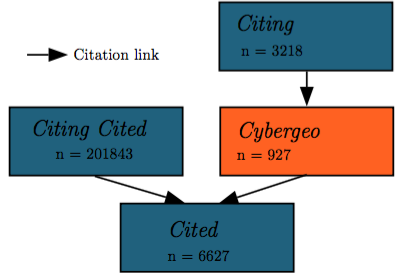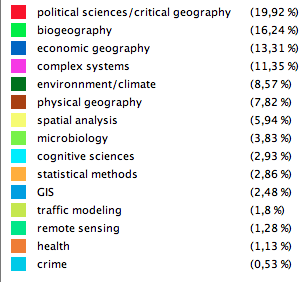# Cybergeo | 1996-2016

Cybergeo turns 20: it’s time to look back for reflection and to anticipate future evolution! cybergeo.revues.org/

### The editorial policy

First entirely electronic journal for social sciences in the world, peer reviewed, European, open (free of charge for authors and readers), with a focus on geography and widely open to the diversity of research agendas and methodologies in all countries. Cybergeo is a success story with now more than one million papers downloaded every year.

### An app to look back

This app builds on 20 years of publication in Cybergeo. You can play with data, drawing geographical networks of authoring, studying and citing through countries, analyzing semantic networks per key words and articles’ content, you can review twenty years of epistemological and thematic trends in a variety of fields of scientific interest. The networks tell who studies what, where and how. Data are regularly updated.

### About the app

All data, materials and source codes are freely available on this repository: github.com/AnonymousAuthor3/cybergeo20#### Time range

scientific articles from authors

authoring countries
Top 5 :

studied countries
Top 5 :

citations from other articles
citations of other articles

#### Data Selection

Search and select a cybergeo paper in the table.

## User guide

### Description

This tab allows to explore the disciplinary neighborhood of cybergeo. It combines citation data with semantic content of articles. The exploration can be at the article level or at an aggregated level with the full semantic network.

### Methodology

#### Citation Data

The citation network is constituted by articles citing cybergeo articles, cited by cybergeo articles, and citing the same articles than cybergeo (citing cited). Structure and number of papers is summed up by the following figure :The data is collected from google scholar (allowing to get only citing articles, thus the particular network structure).

#### Semantic Data

For around 200000 articles of the citation network, abstracts were collected via the Mendeley API. The extraction of significant keywords (n-grams), following [Chavalarias and Cointet, 2013], allows the construction of a semantic network by co-occurrence analysis, which nodes are keywords. Community detection (Louvain algorithm), optimized on filtering parameters (hub filtering and low edge filtering), yield disciplines which are given in the following figure :### How to use

The tab Network Exploration provides article-level exploration. The user can search an article in cybergeo with the datatable. Once one is selected, different informations are displayed if available [note that ~600 papers among 900 have citation and semantic information].

• citation neighborhood of the selected article
• semantic content of the selected article as a colored wordcloud (disciplines legend above), and semantic content for the neighborhood

Graphic options
Graphic options

## User guide

### Description

Exploratory Analysis of Cybergeo Keywords

### Methodology

#### Vertices and nodes attributes

The vertices are described by two variables: frequency and degree. The frequency is the number of articles citing the keyword. The degree is the total degree of the nodes in the network, that is the number of edges linking thiw keyword to the others (there is no distinction between in- and out- degree as the network is undirected). Both variables are distinct but correlated.

The edges are described by two variables: observed weight and relative residual. For two given keywords the observed weight is the number of articles citing both keywords. The relative residual is the ratio between the observed weight and the expected weigth of the edge. For a given edge the expected weight is the probability that this edge exists considering the degree of the nodes. It is computed as the union of two dependant probabilities.

The probability of drawing a vertex i equals $\frac{{w}_{i}}{w}$${w}_{i}$ is the degree of vertex i (weighted degree) and w the half sum of weights.

Then the probability of drawing a vertex j distinct from i equals $\frac{{w}_{j}}{w-{w}_{i}}$.

The probability of existence of an edge between i and j is:

${P}_{i->j}=\frac{{w}_{i}}{w}×\frac{{w}_{j}}{w-{w}_{i}}$

The probability of existence of an edge between j and i:

${P}_{j->i}=\frac{{w}_{j}}{w}×\frac{{w}_{i}}{w-{w}_{j}}$

The probability of existence of an undirected edge is the union of both probabilities:

${P}_{i<->j}={P}_{i->j}+{P}_{j->i}$

Eventually the expected weight is:

${w}^{e}=w\left(\frac{{P}_{i<->j}}{2}\right)$

#### Algorithme de détection de communautés

The community detection is computed with the Louvain algorithm which finds an optimum of modularity. See Blondel et al. 2008.